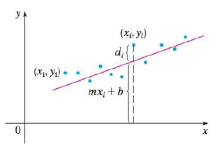Chapter 14.7, Problem 59E

Chapter
Section
Textbook Problem

Suppose that a scientist has reason to believe that two quantities x and y are related linearly, that is, y = mx + b, at least approximately, for some values of m and b. The scientist performs an experiment and collects data in the form of points (x1, x2), (x2, y2) ,…, (xn, yn) and then plots these points. The points don’t lie exactly on a straight line, so the scientist wants to find constants m and b so that the line y = mx + b “fits” the points as well as possible (see the figure).Let di = yi − (mxi, + b) be the vertical deviation of the point (xi, yi) from the line. The method of least squares determines m and b so as to minimize ∑ i = 1 n d i 2 , the sum of the squares of these deviations. Show that, according to this method, the line of best fit is obtained when m ∑ i = 1 n x i + b n = ∑ i = 1 n y i and m ∑ i = 1 n x i 2 + b ∑ i = 1 n x i = ∑ i = 1 n x i y i

To determine

To show: The line of best fit obtained only when mi=1nxi+bn=i=1nyi and mi=1nxi2+bi=1nxi=i=1nxiyi .

Explanation

Given:

Let di=yi(mxi+b) be the vertical deviation of the point (xi,yi) from the line.

The method of least squares determines m and b to minimize i=1ndi2 is the sum of the squares of these deviations.

Proof:

Let di=yi(mxi+b) and to minimize the given equation into i=1ndi2 .

Substitute di=yi(mxi+b) in the function i=1ndi2 .

f(m,b)=i=1n[yi(mxi+b)]2

Note that the above function is a variable of m and b.

Take partial derivative of f(m,b) with respect to m and obtain fm .

fm=m[i=1n[yi(mxi+b)]2]=[i=1n2[yi(mxi+b)]](0xi0)=i=1n2xi[yi(mxi+b)]

Thus, fm=i=1n2xi[yi(mxi+b)] (1)

Set the above partial derivative to zero.

From equation (1),

2i=1nxi[yi(mxi+b)]=0i=1n(xiyimxi2bxi)=0i=1nxiyii=1nmxi2i=1nbxi=0i=1nxiyi=i=1nmxi2+i=1nbxi

Simplify further as follows.

i=1nxiyi=mi=1nxi2+i=1nbxii=1nxiyi=mi=1nxi2+bi=1nxi

Therefore, i=1nxiyi=mi=1nxi2+bi=1nxi is proved.

Take partial derivative of f(m,b) with respect to b and obtain fb .

fb=b[i=1n[yi(mxi+b)]2]=[i=1n2[yi(mxi+b)]](001)=i=1n2[yi(mxi+b)]

Thus, fb=i=1n2[yi(mxi+b)] (2)

Set the above partial derivative to zero.

From equation (2),

2i=1n[yi(mxi+b)]=0i=1n(yimxib)=0i=1nyii=1nmxii=1nb=0i=1nyi=i=1nmxi+i=1nb

Simplify further as follows

Still sussing out bartleby?

Check out a sample textbook solution.

See a sample solution

The Solution to Your Study Problems

Bartleby provides explanations to thousands of textbook problems written by our experts, many with advanced degrees!

Get Started

Use the properties of integrals to verify the inequality. /4/2sinxxdx22

Single Variable Calculus: Early Transcendentals, Volume I

Solve the equations in Exercises 126. (x2+1)x+1(x+1)3=0

Finite Mathematics and Applied Calculus (MindTap Course List)

In Exercises 7-28, perform the indicated operations and simplify each expression. 17. 1+1x11x

Applied Calculus for the Managerial, Life, and Social Sciences: A Brief Approach

Evaluate in problems 1-4. Write all the answers without using exponents. 1. (a) ...

Mathematical Applications for the Management, Life, and Social Sciences

The harmonic series is: 1 + 2 + 3 + 4 + …

Study Guide for Stewart's Single Variable Calculus: Early Transcendentals, 8th

For , f(x) = 0 2 3 f(3) does not exist

Study Guide for Stewart's Multivariable Calculus, 8th